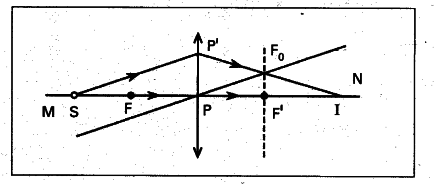# Draw a ray diagram to locate the position

Draw a ray diagram to locate the position of image when a point source (S) is placed on optical axis MN of a convex lens, in such a way that it is beyond focal point (F). See figure.

1. Draw a perpendicular line to principal axis passing the focus (F).
2. Draw a ray from point source (S) in any direction to meet lens at point §.1. Now draw another line parallel to the ray drawn from the point source (S) and passes through the pole §. This line intersect the normal at point F _{ 0 }.
2. Now draw a line passing from point P’ to pass through the point F _{ 0 } such that it meets principal axis at a point (say I).
3. T is the image point for the point source (S).